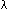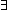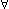The Eladr Protocol is a decentralized, security and efficiency enhanced Web3 noSQL database powered by IPFS as the data storage layer https://ipfs.io/, and the Cardano block chain as the rewards token platform, https://cardano.org/. It provides a JSON based, IPFS layer 2 solution for data indexing and retrieval in an 'append only' file system built with open source Node.js API libraries.

The ELADR token was designed to incentivize and reward community members as a proof of contribution. Token holders are also granted access to EduLadder.com premium features as well as associated ELADR token enabled apps.

WHITE PAPER Buy Now Try BETA

Real Problems! Real Experts!

Watch related videos of your favorite subject.
Connect with students from different parts of the world.
See Our team
Wondering how we keep quality?
Got unsolved questions?

Digital-circuit-and-logic-design-->View question

## What is undecidability of predicate logic?

##### in terms of logic of computers

Taged users:

Likes:
Be first to like this question

Dislikes:
Be first to dislike this question

Theorem: It is undecidable whether a first order logic formula is provable (or true under all possible interpretations).

Proof: Suppose there is an algorithm B that, given a first order logic and a formula in that logic, decides whether that formula is valid (holds under all possible interpretations). I will use that to give a decision algorithm for the language {(M,w) | M is the description of a Turing machine that accepts the string w}. As the latter problem is undecidable this will show that B cannot exists.

Given M and w, create a first order logic by declaring a constant, a unary function symbol a for every letter a in the alphabet, and a binary predicate fq for every state q of M.

Consider the following interpretation of this logic: Variables x range over strings over the given alphabet,denotes the empty string, a(w) denotes the string aw, and fq(x,y) indicates that M, when given input w, can reach a configuration with state q, in which xy is on the tape, with x in reverse order, and the head of M points at the first position of y. Under this interpretation fq0(,w) is certainly a true formula, as the initial configuration is surely reachable. Here q0 is the initial state, and w is a representation of w made from the constant and function symbols of the logic. Furthermore the formulay: fq-acc(x,y) with q-acc the acceptance state, holds iff M accepts w.

Whenever M has a transition from state q to state r, reading a, writing b, and moving right, the formulay: fq(x,ay) => fr(bx,y)

holds. Here x and y are variables. Likewise, if M has a transition from state q to state r, reading a, writing b, and moving left, the formulasy: fq(cx,ay) => fr(x,cby)

hold for every choice of a letter c. In addition we havey: fq(,ay) => fr(,by),

covering the case that M cannot move left, because its head is already in the left-most position. Finally, there are variants of the formulas above for the case that a is the blank symbol and that square of the tape is visited for the first time:y: fq(x,) => fr(bx,y: fq(cx,) => fr(x,cby: fq(,) => fr(,b).

Let T be the conjunction of all implication formulas mentioned above. As M has finitely many transitions and the alphabet is finite, this conjunction is finite as well, and thus a formula of first order logic. Now consider the formula

fq0(,w) & T =>y: fq-acc(x,y).

In case M accepts w, there is a valid computation leading to an accept state. Each step therein corresponds with a substitution instance of one of the conjuncts in T, and using the laws of first order logic it is easy to check that the formula above is provable and thus true under all interpretations. If, on the other hand, the formula above is true under all interpretations, it is surely true in the given interpretation, which implies that M has an accepting computation starting on w.

Thus, in order to decide whether or not M accepts w, it suffices to check whether or not the formula above is

Likes:
Be first to like this answer

Dislikes:
Be first to dislike this answer